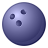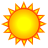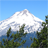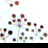# Re: Polygon Width

17623
14
12-15-2011 08:07 AMNew Contributor II
Hi there,

I'm having a problem calculating polygon width. Essentially I have a number of polygons (1000+) that are all irregular, majority trapezoids but some others that probably don't even constitute a shape. What i want to do is find out the width of the polygons at their base (lets call this the shortest width of the trapezoid) and at their top (the largest width of the trapezoid).

Any ideas on how I could go about this? Attached is a picture of what I'm dealing with here:
Tags (3)
14 RepliesNew Contributor II
Example of polygons I have to calculate width for:byMVP Legendary Contributor
If you have ArcGIS 10, then use bounding containers with the extent rectangle option, this will provide the base information that you need.
http://resources.arcgis.com/gallery/file/geoprocessing/details?entryID=3D230972-1422-2418-34A5-2F3FF...Regular Contributor III
You could probably even get the base width using a Python expression in the field calculator.

See this: http://help.arcgis.com/en/arcgisdesktop/10.0/help/index.html#//002z0000001s000000

The "built-in" .hullrectangle geometry property gives you the coordinate pairs of the corners of the convex hull rectangle that encompasses the extent of a polygon. Think of it as an extent polygon that is tilted in such a way to minimize the extent area.

The !Shape.hullRectangle! expression returns a string like:

611210.287367493 1143140.09588692 611179.291388619 1143388.50437016 611781.906192586 1143463.69759822 611812.90217146 1143215.28911498

Where the 1st and 2nd coordinate pairs "seem" to always describe the WIDTH (not the length) of the rectangle, which would be your maximum base width. What's that Pythag. theorem again?

Getting the minimum base width (the skinny part at the top of your trapezoids) would be quite tricky. However, if there are always only 4 coordinate that make up the trapezoid shape, well then that would be easy.New Contributor II
Hi Dan,

While the bounding tool did work on normal polygons, as you can see from the attached images, the irregularity of the ones I am dealing with, did not really work out:

I tried convex hull, which did work, but did not provide the shortaxis etc that the bounding with rectangle did.

Any ideas?New Contributor II
Hi Chris,

The tricky part as you identified is figuring out the skinny part of the trapezoid, and many of my polygons also don't have just the 4 coordinates... It's been giving me a real headache. I'm going to have a swing at the python you showed me though. See if it yields any results. I'll let you know how it goes.

Cheers,
JontyRegular Contributor III
I wonder if you could simplify all the polygons that had > 4 verticies to the point where they had only four constitient verticies (maybe uysing the SimplifyPolygon tool). Probably have to be scripted to keep checking/adjusting the tolerances and resulting vetex counts (feature by feature) untill you get the magic count of four verticies.

Then examining the length of the (now exactly 4 sided) polygon edges, where the two shortest edges will be your min/max base widths (aka the trapezoid top and bottom).byEsri Regular Contributor
If each of your trapezoids has just four corners, or if you can use Simplify Polygon to get rid of extra vertices, then you can try the following (ArcInfo licensing):
1. Run the Minimum Bounding Geometry tool RECTANGLE_BY_WIDTH to get bounding rectangles from the trapezoids; specify to output the characteristic attributes. The resulting MBG_Width values would be the larger base of the trapezoids (assuming the bases are shorter than the sides).
2. Use Split Line At Vertices to get four lines from each trapezoid or rectangle; each line carrries its ORIG_FID (original polygon id).
3. Use Summary Statistics to get the Minimum value of the shape_length, using the ORIG_FID as the Case Field. This should give you the shorter base width for each trapezoid.

Does that help?Regular Contributor III
Would you entertain a method that would give approximate widths (say within 10ft) of the true width?Occasional Contributor III
One genreally accepted way of measuring irregular polygons is to get the extream length, and then find the perpendicular width half way between the ends. That is how I approached the problem for a system I built a couple years ago.

I took the polygon, and generated a near table for points created from all the verticies. I then found the maximum length, and the pair of points to which that length referred.
I genereated a line between these points (the length of the polygon), then rotated the line 90° and clipped it with the polygon. The length of that clipped line is the width.

With this method, the length is exact, and is the greatest overall length. The width is a conventional width. It worked for me because I was most concerned with the extream length and was content to approximate the width.

You could use the length line (before rotation) to perform another near distance calculation to find the polygon vertex points furthest from that line in either direction. Adding the two distances (furthest distance to the left and furthest to the right) gives the overall width of the polygon, square to the longest dimension line.